Schubert calculus

(diff) ← Older revision | Latest revision (diff) | Newer revision → (diff)

Schubert enumerative calculus

A formal calculus of symbols representing geometric conditions used to solve problems in enumerative geometry. This originated in work of M. Chasles [a3] on conics and was systematized and used to great effect by H. Schubert in [a13]. The justification of Schubert's enumerative calculus and the verification of the numbers he obtained was the contents of Hilbert's 15th problem (cf. also Hilbert problems).

Justifying Schubert's enumerative calculus was a major theme of twentieth century algebraic geometry, and intersection theory provides a satisfactory modern framework. Enumerative geometry deals with the second part of Hilbert's problem. See [a7] for a complete reference on intersection theory; for historical surveys and a discussion of enumerative geometry, see [a9], [a10].

The Schubert calculus also refers to mathematics arising from the following class of enumerative geometric problems: Determine the number of linear subspaces of projective space that satisfy incidence conditions imposed by other linear subspaces. For a survey, see [a12]. For example, how many lines in projective-space meetgiven lines? These problems are solved by studying both the geometry and the cohomology or Chow rings of Grassmann varieties (cf. also Chow ring; Grassmann manifold). This field of Schubert calculus enjoys important connections not only to algebraic geometry and algebraic topology, but also to algebraic combinatorics, representation theory, differential geometry, linear algebraic groups, and symbolic computation, and has found applications in numerical homotopy continuation [a8], linear algebra [a6] and systems theory [a2].

The Grassmannian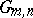of-dimensional subspaces (-planes) in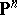over a fieldhas distinguished Schubert varietieswhereis a flag of linear subspaces with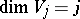. The Schubert cycle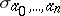is the cohomology class Poincaré dual to the fundamental homology cycle of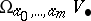(cf. also Homology). The basis theorem asserts that the Schubert cycles form a basis of the Chow ring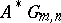(whenis the complex number field, these are the integral cohomology groups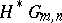) of the Grassmannian with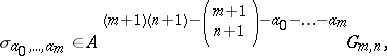(see also Grassmann manifold). The duality theorem asserts that the basis of Schubert cycles is self-dual under the intersection pairing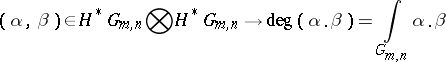withdual to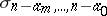.

Let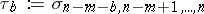be a special Schubert cycle (cf. Schubert cycle). Then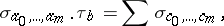the sum running over allwithand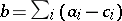. This Pieri formula determines the ring structure of cohomology; an algebraic consequence is the Giambelli formula for expressing an arbitrary Schubert cycle in terms of special Schubert cycles. Define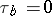if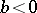or, and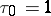. Then Giambelli's formula is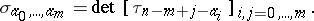These four results enable computation in the Chow ring of the Grassmannian, and the solution of many problems in enumerative geometry. For instance, the number of-planes meeting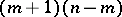general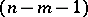-planes non-trivially is the coefficient ofin the product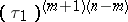, which is [a14]These four results hold more generally for cohomology rings of flag manifolds; Schubert cycles form a self-dual basis, the Chevalley formula [a4] determines the ring structure (whenis a Borel subgroup), and the Bernshtein–Gel'fand–Gel'fand formula [a1] and Demazure formula [a5] give the analogue of the Giambelli formula. More explicit Giambelli formulas are provided by Schubert polynomials.

One cornerstone of the Schubert calculus for the Grassmannian is the Littlewood–Richardson rule [a11] for expressing a product of Schubert cycles in terms of the basis of Schubert cycles. (This rule is usually expressed in terms of an alternative indexing of Schubert cycles using partitions. A sequence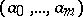corresponds to the partition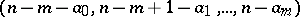; cf. Schur functions in algebraic combinatorics.) The analogue of the Littlewood–Richardson rule is not known for most other flag varieties.

How to Cite This Entry:
Schubert calculus. Encyclopedia of Mathematics. URL: http://encyclopediaofmath.org/index.php?title=Schubert_calculus&oldid=15371
This article was adapted from an original article by Frank Sottile (originator), which appeared in Encyclopedia of Mathematics - ISBN 1402006098. See original article